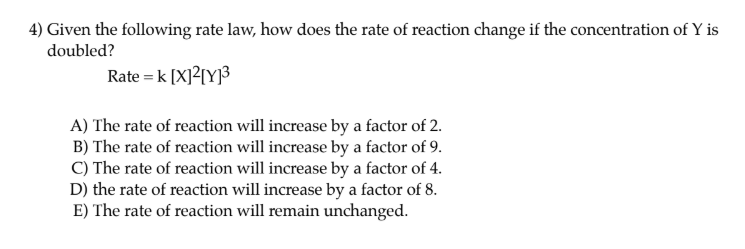# 4) Given the following rate law, how does the rate of reaction change if the concentration of Y is doubled? Rate = k [X]2[Y]3 A) The rate of reaction will increase by a factor of 2. B) The rate of reaction will increase by a factor of 9. C) The rate of reaction will increase by a factor of 4. D) the rate of reaction will increase by a factor of 8. E) The rate of reaction will remain unchanged.